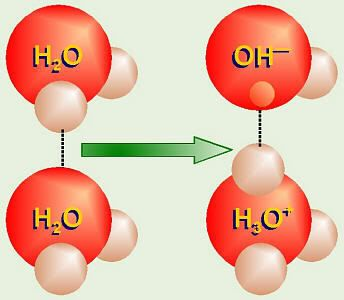pH

The pH of a solution is affected by the concentration of H3O+ (hydronium ion which is often written as H+) and OH. Even in distilled water, one of the hydrogen protons from one water molecule might “jump over” to one of the pairs of unshared electrons in another water molecules and leaving one electron behind causing the formation of H3O+ and OH. This reaction can be written as:2H2O -> H3O+ + OH

The concentration of H3O+ and OH in pure distilled water is 1 × 10–7 mol/dm³ each. However, if other substances are added, the concentration of H+ and OH– might change. If the concentration of H+ is more than 1 × 10–7 mol/dm³, the solution is acidic, if the concentration of H+ is less than 1 × 10–7 mol/dm³, the solution is basic. It should also be noted that the multiplication of the concentration of both H+ and OH is always 10–14. So if one increases, the other decreases.

The pH of a solution is the measure of the concentration of H+ in one dm³ of that solution. For example, if the concentration of H+ is 1 × 10–5 mol/dm³, the pH is equals to 5. Hence it is obvious that pH is on a logarithm scale, which means that pH 4 is actually ten times more acidic than pH 5.

An acid is a substance which adds H+ to a solution. A base is a substance which subtracts H+ from or adds OH to a solution. A solution with pH of 7 is known as neutral solution. Some examples of acidic substance are lemon juice or vinegar with pH of around 3, and the hydrochloric acid in our stomach is between 1 to 3 pH. Lye (NaOH), the main ingredient in many drain-openers, is a strong base, with a pH of 12 to 14.

page revision: 9, last edited: 18 Sep 2007 15:47